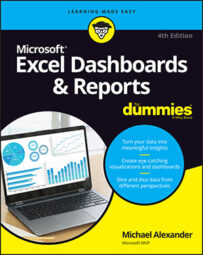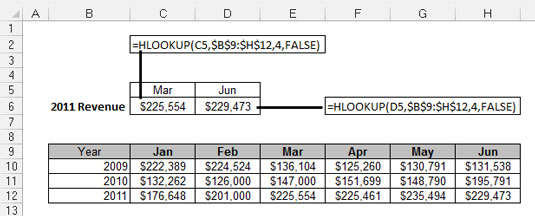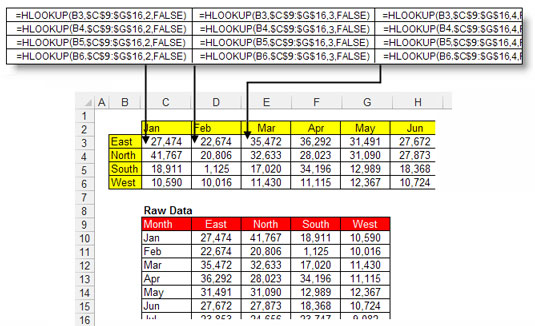##### Excel Dashboards & Reports For DummiesThe HLOOKUP function in Excel is the less popular cousin of the VLOOKUP function. The H in HLOOKUP stands for horizontal. Because Excel data is typically vertically oriented, most situations require a vertical lookup, or VLOOKUP.

However, some data structures are horizontally oriented, requiring a horizontal lookup; thus, the HLOOKUP function comes in handy. The HLOOKUP searches a lookup table to find a single value from a row of data where the column label matches a given criterion.

## HLOOKUP basics

The following figure demonstrates a typical scenario in which HLOOKUP formulas are used. The table in C5 requires quarter-end numbers (March and June) for 2011. The HLOOKUP formulas use the column labels to find the correct month columns and then locate the 2011 data by moving down the appropriate number of rows. In this case, 2011 data is in row 4, so the number 4 is used in the formulas.HLOOKUP formulas help to find March and June numbers from the lookup tabl" width="535"/>
HLOOKUP formulas help to find March and June numbers from the lookup table.

To get your mind around how this works, take a look at the basic syntax of the HLOOKUP function.

`HLOOKUP(Lookup_value, Table_array, Row_index_num, Range_lookup)`
• Lookup_value: The Lookup_value argument identifies the value being looked up. In most cases, these values are column names. In the example, the column labels are being referenced for the Lookup_value. This points the HLOOKUP function to the appropriate column in the lookup table.

• Table_array: The Table_array argument identifies the range that contains the lookup table. Here, that range is B9:H12. Notice that the references used for this argument are absolute. This means the column and row references are prefixed with dollar (\$) signs — as in \$B\$9:\$H\$12. This ensures that the reference doesn't shift while you copy the formula down or across.

• Row_index_num: The Row_index_num argument identifies the row number that contains the value you're looking for. In the example, the 2011 data is located in row 4 of the lookup table. Therefore, the formulas use the number 4.

• Range_lookup: The Range_lookup argument specifies whether you're looking for an exact match or an approximate match. If an exact match is needed, you'd enter FALSE for this argument. If the closest match will do, you'd enter TRUE or leave the argument blank.

## Applying HLOOKUP formulas in a data model

HLOOKUPs are especially handy for shaping data into structures appropriate for charting or other types of reporting. A simple example is demonstrated in the following figure. With HLOOKUPs, the data shown in the raw data table at the bottom of the figure is reoriented in a staging table at the top. When the raw data is changed or refreshed, the staging table captures the changes.HLOOKUP formulas pull and reshape data without disturbin" width="535"/>
In this example, HLOOKUP formulas pull and reshape data without disturbing the raw data table.